# Exploratory Data Analysis in Python

For data analysis, Exploratory Data Analysis (EDA) must be your first step. Exploratory Data Analysis helps us to −

• To give insight into a data set.

• Understand the underlying structure.

• Extract important parameters and relationships that hold between them.

• Test underlying assumptions.

## Understanding EDA using sample Data set

To understand EDA using python, we can take the sample data either directly from any website or from your local disk. I’m taking the sample data from the UCI Machine Learning Repository which is publicly available of a red variant of Wine Quality data set and try to grab much insight into the data set using EDA.

import pandas as pd
df.head()

Running above script in jupyter notebook, will give output something like below −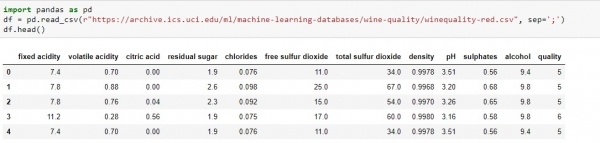• Firstly, import the necessary library, pandas in the case.

• Read the csv file using read_csv() function of pandas library and each data is separated by the delimiter “;” in given data set.

• Return the first five observation from the data set with the help of “.head” function provided by the pandas library. We can get last five observation similarly by using the “.tail()” function of pandas library.

We can get the total number of rows and columns from the data set using “.shape” like below −

df.shape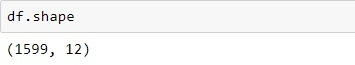To find what all columns it contains, of what types and if they contain any value in it or not, with the help of info() function.

df.info()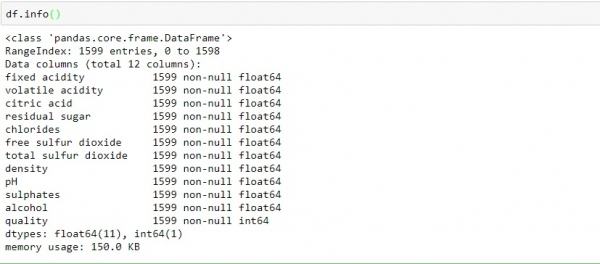By observing the above data, we can conclude −

• Data contain an only float an integer value.

• All the columns variable are non-null (no-empty or missing value).

Another useful function provided by pandas is describe() which provides the count, mean, standard deviation, minimum and maximum values and the quantities of the data.

df.describe()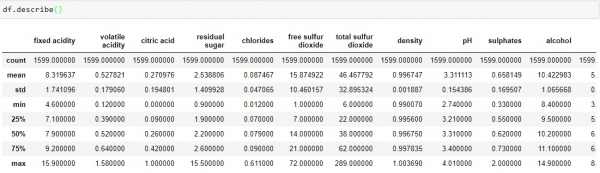• From above data, we can conclude that the mean value of each columns is less than the median value (50%) in index column.

• There is a huge difference between the 75% and max values of predictors “residual sugar”, “free sulfur dioxide” and “total sulfur dioxide”.

• Above two observations, gives an indication that there are extreme values- deviations in our data set.

Couples of key insights we can get from dependent variables are as follow −

df.quality.unique()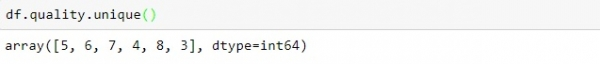• In “quality” score scale, 1 comes at the bottom .i.e. poor and 10 comes at the top .i.e. best.

• From above we can conclude, none of the observation score 1(poor), 2 and 9, 10(best) score. All the scores are between 3 to 8.

df.quality.value_counts()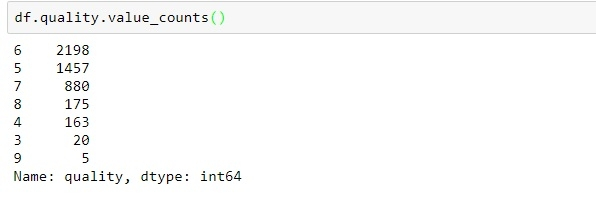• Above processed data provide an information on vote count for each quality score in descending order.

• Most of the quality are in the range of 5-7.

• Least observations are observed in the 3 and 6 categories.

## Data Visualizations

To check Missing Values −

We can check missing values in our white-whiskey csv data set with the help of seaborn library. Below is the code to fullfil that −

import pandas as pd
import numpy as np
import seaborn as sns
import matplotlib.pyplot as plt
%matplotlib inline
sns.set()
sns.heatmap(df.isnull(), cbar=False, yticklabels=False, cmap='viridis')

## Output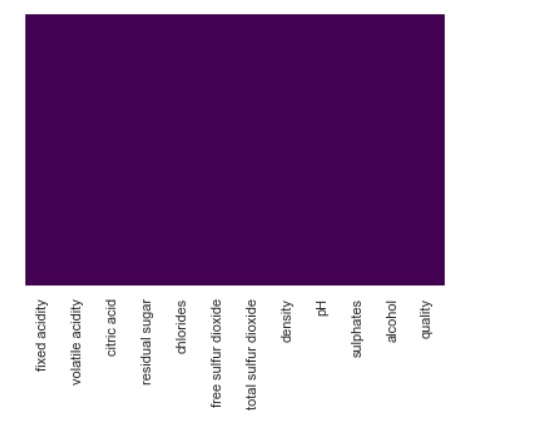• From above we can see there is no missing values in the dataset. Incase if there is any, we would have seen figure represented by different colour shade on purple background.

• With different dataset where there are missing values and you’ll notice the difference.

## To check correlation

To check correlation between different values of the dataset, insert below code in our existing dataset −

plt.figure(figsize=(8,4))
sns.heatmap(df.corr(),cmap='Greens',annot=False)

## Output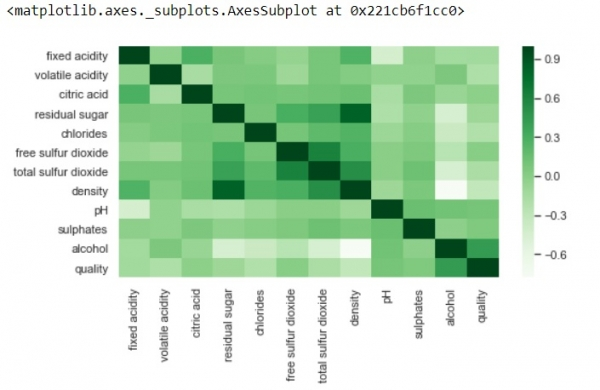• Above, positive correlation is represented by dark shades and negative correlation by lighter shades.

• Changes the value of annot=True, and the output will show you values by which features are correlated to each other in grid-cells.

We can generate another correlation matrix with annot=True. Modify your code by adding below lines of code to our existing code −

k = 12
cols = df.corr().nlargest(k, 'quality')['quality'].index
cm = df[cols].corr()
plt.figure(figsize=(8,6))
sns.heatmap(cm, annot=True, cmap = 'viridis')

## Output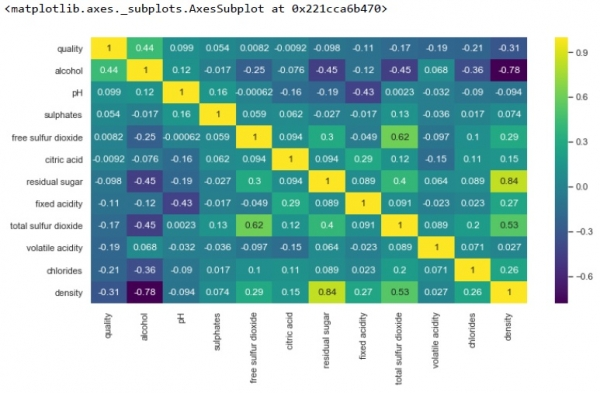• From above we can see, there is a strong positive correlation of density with residual sugar. However, a strong negative correlation of density and alcohol.

• Also, there is no correlation between free sulphur dioxide and quality.